# Mean State

Period Mean (original grids) [Watt m-2]
Model Period Mean (intersection) [Watt m-2]
Model Period Mean (complement) [Watt m-2]
Benchmark Period Mean (intersection) [Watt m-2]
Benchmark Period Mean (complement) [Watt m-2]
Bias [Watt m-2]
RMSE [Watt m-2]
Phase Shift [months]
Bias Score 
RMSE Score 
Seasonal Cycle Score 
Spatial Distribution Score 
Interannual Variability Score 
Overall Score 
Benchmark [-] 122.
CLM4 [-] 140. 140. 0.00 120. 164. 20.0 28.8 1.53 0.31 0.41 0.79 1.0 0.57 0.58
CLM4.5 [-] 136. 136. 0.00 120. 164. 16.2 27.0 1.56 0.37 0.40 0.78 0.99 0.58 0.59
CLM5 [-] 135. 135. 0.00 120. 164. 15.3 25.4 1.44 0.39 0.41 0.80 0.97 0.55 0.59
Period Mean (original grids) [Watt m-2]
Model Period Mean (intersection) [Watt m-2]
Model Period Mean (complement) [Watt m-2]
Benchmark Period Mean (intersection) [Watt m-2]
Benchmark Period Mean (complement) [Watt m-2]
Bias [Watt m-2]
RMSE [Watt m-2]
Phase Shift [months]
Bias Score 
RMSE Score 
Seasonal Cycle Score 
Spatial Distribution Score 
Interannual Variability Score 
Overall Score 
Benchmark [-] 102.
CLM4 [-] 113. 112. 0.00 101. 165. 11.6 25.8 1.39 0.59 0.45 0.80 0.99 0.66 0.65
CLM4.5 [-] 108. 108. 0.00 101. 165. 7.52 24.3 1.44 0.63 0.45 0.80 0.98 0.66 0.66
CLM5 [-] 108. 107. 0.00 101. 165. 6.68 23.4 1.50 0.65 0.46 0.78 0.99 0.62 0.66
Period Mean (original grids) [Watt m-2]
Model Period Mean (intersection) [Watt m-2]
Model Period Mean (complement) [Watt m-2]
Benchmark Period Mean (intersection) [Watt m-2]
Benchmark Period Mean (complement) [Watt m-2]
Bias [Watt m-2]
RMSE [Watt m-2]
Phase Shift [months]
Bias Score 
RMSE Score 
Seasonal Cycle Score 
Spatial Distribution Score 
Interannual Variability Score 
Overall Score 
Benchmark [-] 112.
CLM4 [-] 117. 117. 0.00 110. 159. 8.28 23.2 1.11 0.63 0.56 0.85 0.97 0.58 0.69
CLM4.5 [-] 112. 111. 0.00 110. 159. 3.69 22.5 1.11 0.65 0.55 0.85 0.95 0.59 0.69
CLM5 [-] 113. 113. 0.00 110. 159. 5.35 22.1 1.18 0.67 0.56 0.84 0.96 0.55 0.69
Period Mean (original grids) [Watt m-2]
Model Period Mean (intersection) [Watt m-2]
Model Period Mean (complement) [Watt m-2]
Benchmark Period Mean (intersection) [Watt m-2]
Benchmark Period Mean (complement) [Watt m-2]
Bias [Watt m-2]
RMSE [Watt m-2]
Phase Shift [months]
Bias Score 
RMSE Score 
Seasonal Cycle Score 
Spatial Distribution Score 
Interannual Variability Score 
Overall Score 
Benchmark [-] 43.3
CLM4 [-] 13.9 14.0 0.00 43.2 44.3 -29.8 36.0 0.644 0.59 0.66 0.96 0.90 0.68 0.74
CLM4.5 [-] 18.2 18.3 0.00 43.2 44.3 -26.3 31.3 0.427 0.63 0.70 0.97 0.78 0.64 0.74
CLM5 [-] 14.6 14.7 0.00 43.2 44.3 -29.9 33.5 0.368 0.59 0.70 0.98 0.76 0.64 0.73
Period Mean (original grids) [Watt m-2]
Model Period Mean (intersection) [Watt m-2]
Model Period Mean (complement) [Watt m-2]
Benchmark Period Mean (intersection) [Watt m-2]
Benchmark Period Mean (complement) [Watt m-2]
Bias [Watt m-2]
RMSE [Watt m-2]
Phase Shift [months]
Bias Score 
RMSE Score 
Seasonal Cycle Score 
Spatial Distribution Score 
Interannual Variability Score 
Overall Score 
Benchmark [-] 144.
CLM4 [-] 163. 163. 0.00 140. 160. 25.9 34.8 1.68 0.30 0.39 0.75 0.83 0.50 0.53
CLM4.5 [-] 162. 161. 0.00 140. 160. 24.8 33.9 1.65 0.31 0.39 0.76 0.86 0.49 0.53
CLM5 [-] 157. 156. 0.00 140. 160. 20.0 30.5 1.51 0.35 0.39 0.78 0.84 0.48 0.54
Period Mean (original grids) [Watt m-2]
Model Period Mean (intersection) [Watt m-2]
Model Period Mean (complement) [Watt m-2]
Benchmark Period Mean (intersection) [Watt m-2]
Benchmark Period Mean (complement) [Watt m-2]
Bias [Watt m-2]
RMSE [Watt m-2]
Phase Shift [months]
Bias Score 
RMSE Score 
Seasonal Cycle Score 
Spatial Distribution Score 
Interannual Variability Score 
Overall Score 
Benchmark [-] 62.5
CLM4 [-] 48.7 48.6 0.00 62.3 75.7 -13.6 27.3 0.293 0.79 0.67 0.98 0.76 0.65 0.75
CLM4.5 [-] 46.3 46.3 0.00 62.3 75.7 -15.9 29.4 0.271 0.77 0.66 0.98 0.70 0.66 0.74
CLM5 [-] 42.4 42.4 0.00 62.3 75.7 -19.8 31.9 0.344 0.71 0.65 0.98 0.88 0.66 0.75
Period Mean (original grids) [Watt m-2]
Model Period Mean (intersection) [Watt m-2]
Model Period Mean (complement) [Watt m-2]
Benchmark Period Mean (intersection) [Watt m-2]
Benchmark Period Mean (complement) [Watt m-2]
Bias [Watt m-2]
RMSE [Watt m-2]
Phase Shift [months]
Bias Score 
RMSE Score 
Seasonal Cycle Score 
Spatial Distribution Score 
Interannual Variability Score 
Overall Score 
Benchmark [-] 72.7
CLM4 [-] 70.4 70.2 0.00 72.1 94.9 -0.664 29.1 0.706 0.71 0.59 0.93 0.81 0.63 0.71
CLM4.5 [-] 68.9 68.7 0.00 72.1 94.9 -2.05 29.8 0.698 0.70 0.59 0.93 0.82 0.63 0.71
CLM5 [-] 66.3 66.0 0.00 72.1 94.9 -4.76 30.0 0.732 0.71 0.57 0.93 0.89 0.64 0.72
Period Mean (original grids) [Watt m-2]
Model Period Mean (intersection) [Watt m-2]
Model Period Mean (complement) [Watt m-2]
Benchmark Period Mean (intersection) [Watt m-2]
Benchmark Period Mean (complement) [Watt m-2]
Bias [Watt m-2]
RMSE [Watt m-2]
Phase Shift [months]
Bias Score 
RMSE Score 
Seasonal Cycle Score 
Spatial Distribution Score 
Interannual Variability Score 
Overall Score 
Benchmark [-] 109.
CLM4 [-] 126. 126. 0.00 105. 157. 24.5 37.0 0.934 0.41 0.49 0.89 0.99 0.58 0.64
CLM4.5 [-] 122. 122. 0.00 105. 157. 21.4 34.9 0.926 0.45 0.49 0.89 0.99 0.57 0.65
CLM5 [-] 121. 121. 0.00 105. 157. 20.0 33.3 0.825 0.46 0.50 0.92 0.99 0.52 0.65
Period Mean (original grids) [Watt m-2]
Model Period Mean (intersection) [Watt m-2]
Model Period Mean (complement) [Watt m-2]
Benchmark Period Mean (intersection) [Watt m-2]
Benchmark Period Mean (complement) [Watt m-2]
Bias [Watt m-2]
RMSE [Watt m-2]
Phase Shift [months]
Bias Score 
RMSE Score 
Seasonal Cycle Score 
Spatial Distribution Score 
Interannual Variability Score 
Overall Score 
Benchmark [-] 41.8
CLM4 [-] 11.0 11.4 0.00 41.9 41.5 -31.3 34.0 0.385 0.56 0.67 0.97 0.79 0.70 0.73
CLM4.5 [-] 12.6 13.0 0.00 41.9 41.5 -30.0 33.0 0.404 0.57 0.67 0.97 0.72 0.70 0.72
CLM5 [-] 9.84 10.2 0.00 41.9 41.5 -32.9 33.8 0.382 0.54 0.67 0.97 0.71 0.70 0.71
Period Mean (original grids) [Watt m-2]
Model Period Mean (intersection) [Watt m-2]
Model Period Mean (complement) [Watt m-2]
Benchmark Period Mean (intersection) [Watt m-2]
Benchmark Period Mean (complement) [Watt m-2]
Bias [Watt m-2]
RMSE [Watt m-2]
Phase Shift [months]
Bias Score 
RMSE Score 
Seasonal Cycle Score 
Spatial Distribution Score 
Interannual Variability Score 
Overall Score 
Benchmark [-] 96.5
CLM4 [-] 98.7 98.7 0.00 93.2 138. 6.87 22.9 0.598 0.72 0.65 0.95 0.92 0.59 0.75
CLM4.5 [-] 98.5 98.4 0.00 93.2 138. 6.64 22.9 0.621 0.72 0.65 0.95 0.90 0.60 0.74
CLM5 [-] 97.5 97.5 0.00 93.2 138. 5.79 23.2 0.580 0.72 0.64 0.95 0.93 0.60 0.75
Period Mean (original grids) [Watt m-2]
Model Period Mean (intersection) [Watt m-2]
Model Period Mean (complement) [Watt m-2]
Benchmark Period Mean (intersection) [Watt m-2]
Benchmark Period Mean (complement) [Watt m-2]
Bias [Watt m-2]
RMSE [Watt m-2]
Phase Shift [months]
Bias Score 
RMSE Score 
Seasonal Cycle Score 
Spatial Distribution Score 
Interannual Variability Score 
Overall Score 
Benchmark [-] 64.4
CLM4 [-] 64.0 64.0 0.00 61.9 90.5 2.72 25.3 0.317 0.78 0.69 0.98 0.84 0.76 0.79
CLM4.5 [-] 65.5 65.5 0.00 61.9 90.5 4.14 25.4 0.308 0.79 0.68 0.98 0.89 0.77 0.80
CLM5 [-] 60.9 61.0 0.00 61.9 90.5 -0.150 25.5 0.326 0.81 0.67 0.98 0.88 0.74 0.79
Period Mean (original grids) [Watt m-2]
Model Period Mean (intersection) [Watt m-2]
Model Period Mean (complement) [Watt m-2]
Benchmark Period Mean (intersection) [Watt m-2]
Benchmark Period Mean (complement) [Watt m-2]
Bias [Watt m-2]
RMSE [Watt m-2]
Phase Shift [months]
Bias Score 
RMSE Score 
Seasonal Cycle Score 
Spatial Distribution Score 
Interannual Variability Score 
Overall Score 
Benchmark [-] 115.
CLM4 [-] 74.6 74.6 0.00 77.4 133. -0.847 29.1 0.799 0.59 0.56 0.91 0.87 0.57 0.68
CLM4.5 [-] 73.7 73.7 0.00 77.4 133. -1.61 28.6 0.797 0.60 0.56 0.91 0.88 0.57 0.68
CLM5 [-] 71.5 71.5 0.00 77.4 133. -4.18 29.2 0.799 0.59 0.56 0.91 0.86 0.56 0.68
Period Mean (original grids) [Watt m-2]
Model Period Mean (intersection) [Watt m-2]
Model Period Mean (complement) [Watt m-2]
Benchmark Period Mean (intersection) [Watt m-2]
Benchmark Period Mean (complement) [Watt m-2]
Bias [Watt m-2]
RMSE [Watt m-2]
Phase Shift [months]
Bias Score 
RMSE Score 
Seasonal Cycle Score 
Spatial Distribution Score 
Interannual Variability Score 
Overall Score 
Benchmark [-] 54.9
CLM4 [-] 64.2 64.2 0.00 51.3 143. 15.0 32.5 1.48 0.52 0.48 0.80 0.85 0.50 0.60
CLM4.5 [-] 64.8 64.8 0.00 51.3 143. 15.7 32.9 1.51 0.52 0.47 0.80 0.86 0.50 0.60
CLM5 [-] 64.5 64.6 0.00 51.3 143. 15.6 32.9 1.47 0.52 0.47 0.80 0.88 0.50 0.61
Period Mean (original grids) [Watt m-2]
Model Period Mean (intersection) [Watt m-2]
Model Period Mean (complement) [Watt m-2]
Benchmark Period Mean (intersection) [Watt m-2]
Benchmark Period Mean (complement) [Watt m-2]
Bias [Watt m-2]
RMSE [Watt m-2]
Phase Shift [months]
Bias Score 
RMSE Score 
Seasonal Cycle Score 
Spatial Distribution Score 
Interannual Variability Score 
Overall Score 
Benchmark [-] 44.9
CLM4 [-] 19.2 19.0 0.00 44.8 45.6 -27.4 32.4 0.234 0.62 0.70 0.98 0.80 0.65 0.74
CLM4.5 [-] 20.5 20.3 0.00 44.8 45.6 -26.3 31.1 0.217 0.63 0.70 0.99 0.79 0.63 0.74
CLM5 [-] 15.2 15.0 0.00 44.8 45.6 -31.6 35.1 0.181 0.57 0.70 0.99 0.75 0.64 0.72
Period Mean (original grids) [Watt m-2]
Model Period Mean (intersection) [Watt m-2]
Model Period Mean (complement) [Watt m-2]
Benchmark Period Mean (intersection) [Watt m-2]
Benchmark Period Mean (complement) [Watt m-2]
Bias [Watt m-2]
RMSE [Watt m-2]
Phase Shift [months]
Bias Score 
RMSE Score 
Seasonal Cycle Score 
Spatial Distribution Score 
Interannual Variability Score 
Overall Score 
Benchmark [-] 134.
CLM4 [-] 117. 117. 0.00 127. 169. -2.29 26.7 1.12 0.59 0.58 0.86 0.97 0.60 0.70
CLM4.5 [-] 118. 118. 0.00 127. 169. -0.859 28.6 1.16 0.55 0.57 0.86 0.94 0.61 0.68
CLM5 [-] 116. 116. 0.00 127. 169. -2.92 27.2 1.04 0.57 0.59 0.88 0.96 0.57 0.69
Period Mean (original grids) [Watt m-2]
Model Period Mean (intersection) [Watt m-2]
Model Period Mean (complement) [Watt m-2]
Benchmark Period Mean (intersection) [Watt m-2]
Benchmark Period Mean (complement) [Watt m-2]
Bias [Watt m-2]
RMSE [Watt m-2]
Phase Shift [months]
Bias Score 
RMSE Score 
Seasonal Cycle Score 
Spatial Distribution Score 
Interannual Variability Score 
Overall Score 
Benchmark [-] 99.6
CLM4 [-] 98.2 98.6 0.00 97.8 136. 2.49 24.7 0.466 0.81 0.64 0.97 0.81 0.62 0.75
CLM4.5 [-] 96.1 96.5 0.00 97.8 136. 0.486 24.8 0.425 0.82 0.64 0.97 0.79 0.62 0.75
CLM5 [-] 89.4 89.7 0.00 97.8 136. -6.20 25.6 0.491 0.81 0.63 0.96 0.81 0.58 0.74
Period Mean (original grids) [Watt m-2]
Model Period Mean (intersection) [Watt m-2]
Model Period Mean (complement) [Watt m-2]
Benchmark Period Mean (intersection) [Watt m-2]
Benchmark Period Mean (complement) [Watt m-2]
Bias [Watt m-2]
RMSE [Watt m-2]
Phase Shift [months]
Bias Score 
RMSE Score 
Seasonal Cycle Score 
Spatial Distribution Score 
Interannual Variability Score 
Overall Score 
Benchmark [-] 72.2
CLM4 [-] 61.3 61.5 0.00 72.0 77.2 -10.8 24.9 0.383 0.83 0.69 0.97 0.88 0.61 0.78
CLM4.5 [-] 61.5 61.6 0.00 72.0 77.2 -10.6 24.4 0.391 0.83 0.69 0.97 0.93 0.61 0.79
CLM5 [-] 54.7 54.9 0.00 72.0 77.2 -17.2 29.2 0.370 0.74 0.66 0.97 0.96 0.61 0.77
Period Mean (original grids) [Watt m-2]
Model Period Mean (intersection) [Watt m-2]
Model Period Mean (complement) [Watt m-2]
Benchmark Period Mean (intersection) [Watt m-2]
Benchmark Period Mean (complement) [Watt m-2]
Bias [Watt m-2]
RMSE [Watt m-2]
Phase Shift [months]
Bias Score 
RMSE Score 
Seasonal Cycle Score 
Spatial Distribution Score 
Interannual Variability Score 
Overall Score 
Benchmark [-] 81.6
CLM4 [-] 68.2 68.7 0.00 81.1 116. -11.7 23.0 0.511 0.75 0.69 0.96 0.98 0.64 0.79
CLM4.5 [-] 66.3 66.8 0.00 81.1 116. -13.5 24.3 0.497 0.73 0.69 0.96 0.97 0.64 0.78
CLM5 [-] 64.3 64.9 0.00 81.1 116. -15.5 26.1 0.532 0.70 0.68 0.96 0.99 0.63 0.77
Period Mean (original grids) [Watt m-2]
Model Period Mean (intersection) [Watt m-2]
Model Period Mean (complement) [Watt m-2]
Benchmark Period Mean (intersection) [Watt m-2]
Benchmark Period Mean (complement) [Watt m-2]
Bias [Watt m-2]
RMSE [Watt m-2]
Phase Shift [months]
Bias Score 
RMSE Score 
Seasonal Cycle Score 
Spatial Distribution Score 
Interannual Variability Score 
Overall Score 
Benchmark [-] 132.
CLM4 [-] 154. 154. 0.00 131. 178. 24.1 30.9 1.10 0.27 0.41 0.85 0.90 0.52 0.56
CLM4.5 [-] 152. 152. 0.00 131. 178. 21.7 29.1 1.11 0.31 0.41 0.85 0.94 0.52 0.57
CLM5 [-] 151. 151. 0.00 131. 178. 20.5 27.7 1.16 0.33 0.42 0.84 0.92 0.51 0.57
Period Mean (original grids) [Watt m-2]
Model Period Mean (intersection) [Watt m-2]
Model Period Mean (complement) [Watt m-2]
Benchmark Period Mean (intersection) [Watt m-2]
Benchmark Period Mean (complement) [Watt m-2]
Bias [Watt m-2]
RMSE [Watt m-2]
Phase Shift [months]
Bias Score 
RMSE Score 
Seasonal Cycle Score 
Spatial Distribution Score 
Interannual Variability Score 
Overall Score 
Benchmark [-] 117.
CLM4 [-] 127. 126. 0.00 117. 145. 10.3 24.7 0.910 0.63 0.53 0.89 0.94 0.59 0.69
CLM4.5 [-] 123. 123. 0.00 117. 145. 7.33 23.1 0.870 0.69 0.54 0.90 0.96 0.60 0.71
CLM5 [-] 121. 121. 0.00 117. 145. 5.09 21.7 1.01 0.71 0.55 0.88 0.93 0.55 0.69
Period Mean (original grids) [Watt m-2]
Model Period Mean (intersection) [Watt m-2]
Model Period Mean (complement) [Watt m-2]
Benchmark Period Mean (intersection) [Watt m-2]
Benchmark Period Mean (complement) [Watt m-2]
Bias [Watt m-2]
RMSE [Watt m-2]
Phase Shift [months]
Bias Score 
RMSE Score 
Seasonal Cycle Score 
Spatial Distribution Score 
Interannual Variability Score 
Overall Score 
Benchmark [-] 95.7
CLM4 [-] 84.1 83.8 0.00 92.5 124. -8.67 26.8 0.560 0.72 0.66 0.95 0.94 0.52 0.74
CLM4.5 [-] 82.4 82.0 0.00 92.5 124. -10.2 27.7 0.601 0.71 0.66 0.94 0.92 0.52 0.74
CLM5 [-] 81.9 81.5 0.00 92.5 124. -10.7 27.6 0.604 0.71 0.66 0.94 0.91 0.52 0.73

# Temporally integrated period mean

BENCHMARK MEAN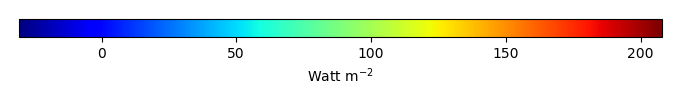MODEL MEANBIAS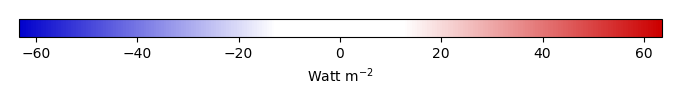BIAS SCORERMSE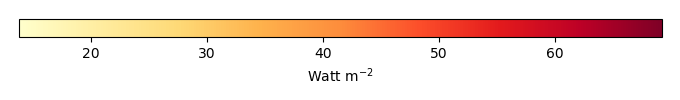RMSE SCOREBENCHMARK INTERANNUAL VARIABILITY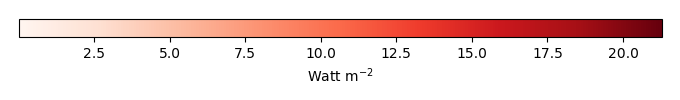MODEL INTERANNUAL VARIABILITYINTERANNUAL VARIABILITY SCOREBENCHMARK MAX MONTHMODEL MAX MONTHDIFFERENCE IN MAX MONTH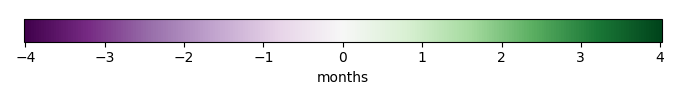SEASONAL CYCLE SCORESPATIAL TAYLOR DIAGRAMMODEL COLORS# Spatially integrated regional mean

MODEL COLORSREGIONAL MEANANNUAL CYCLEMONTHLY ANOMALYANNUAL CYCLE# All Models

BenchmarkCLM4CLM4.5CLM5# Data Information

creation_date: Tue Jul 1 08:16:58 PDT 2014

source_file: This product is generated from monthly 1 degree GEWEX SRB Radiation observations